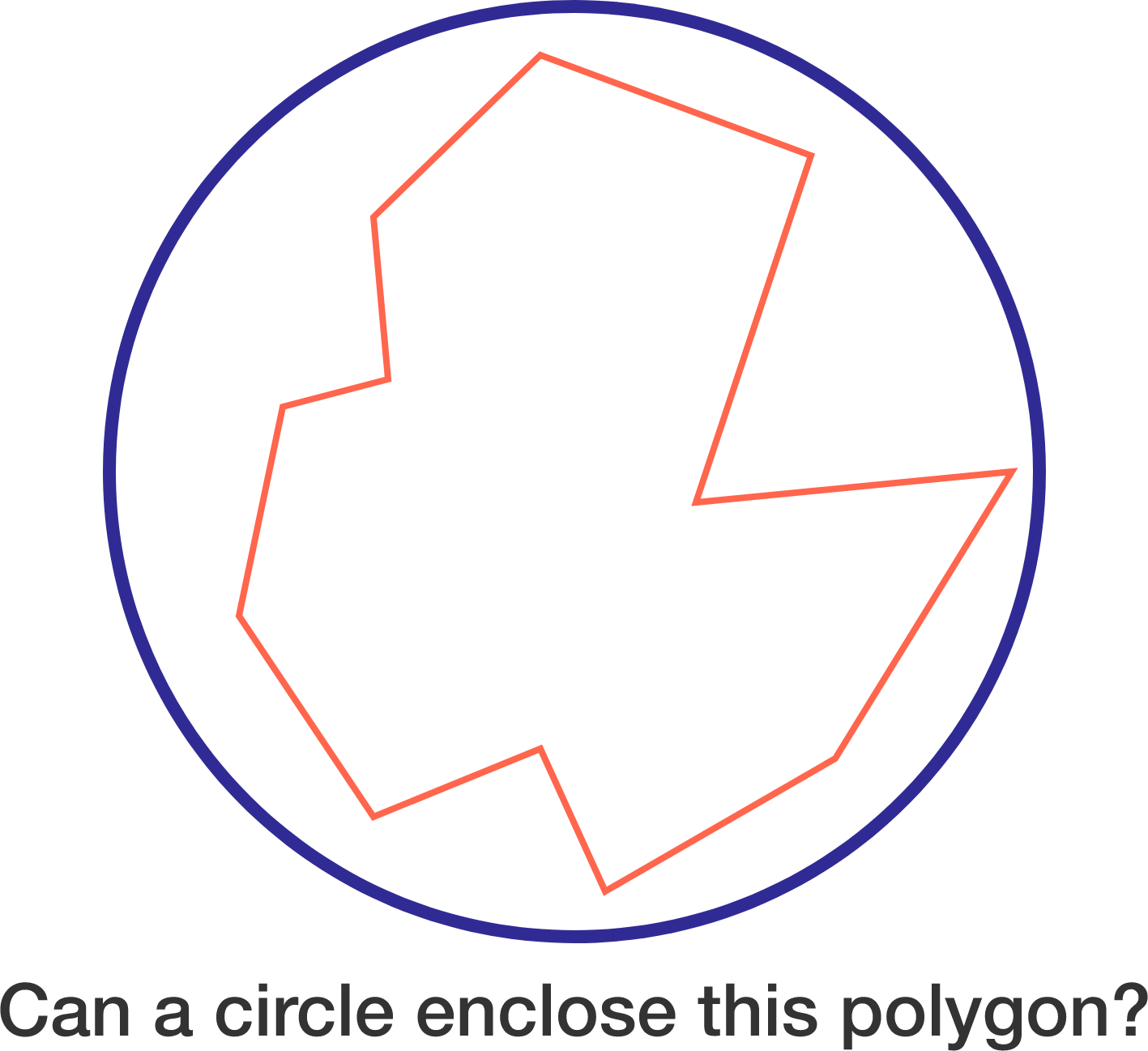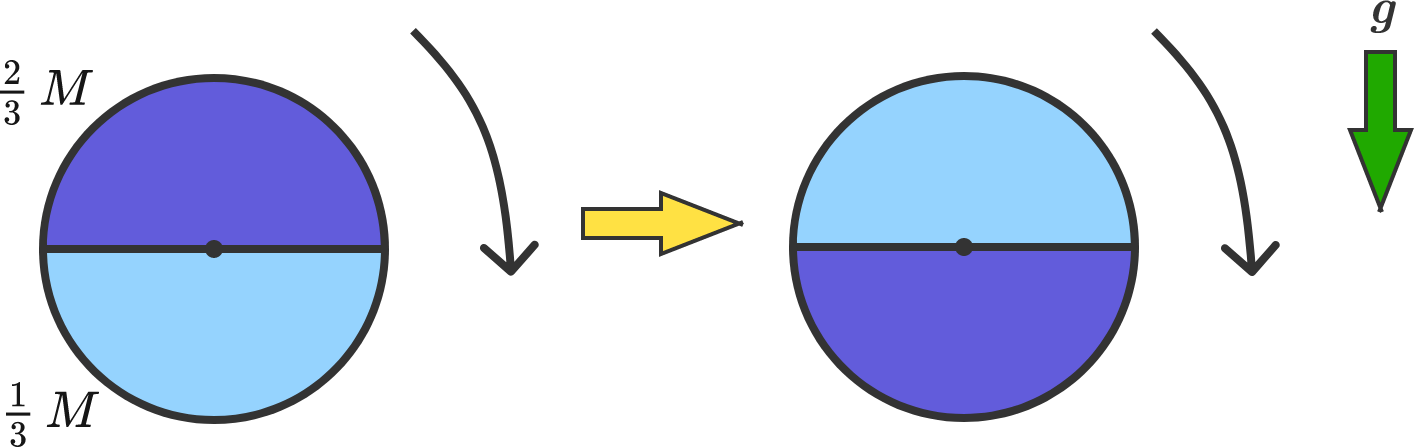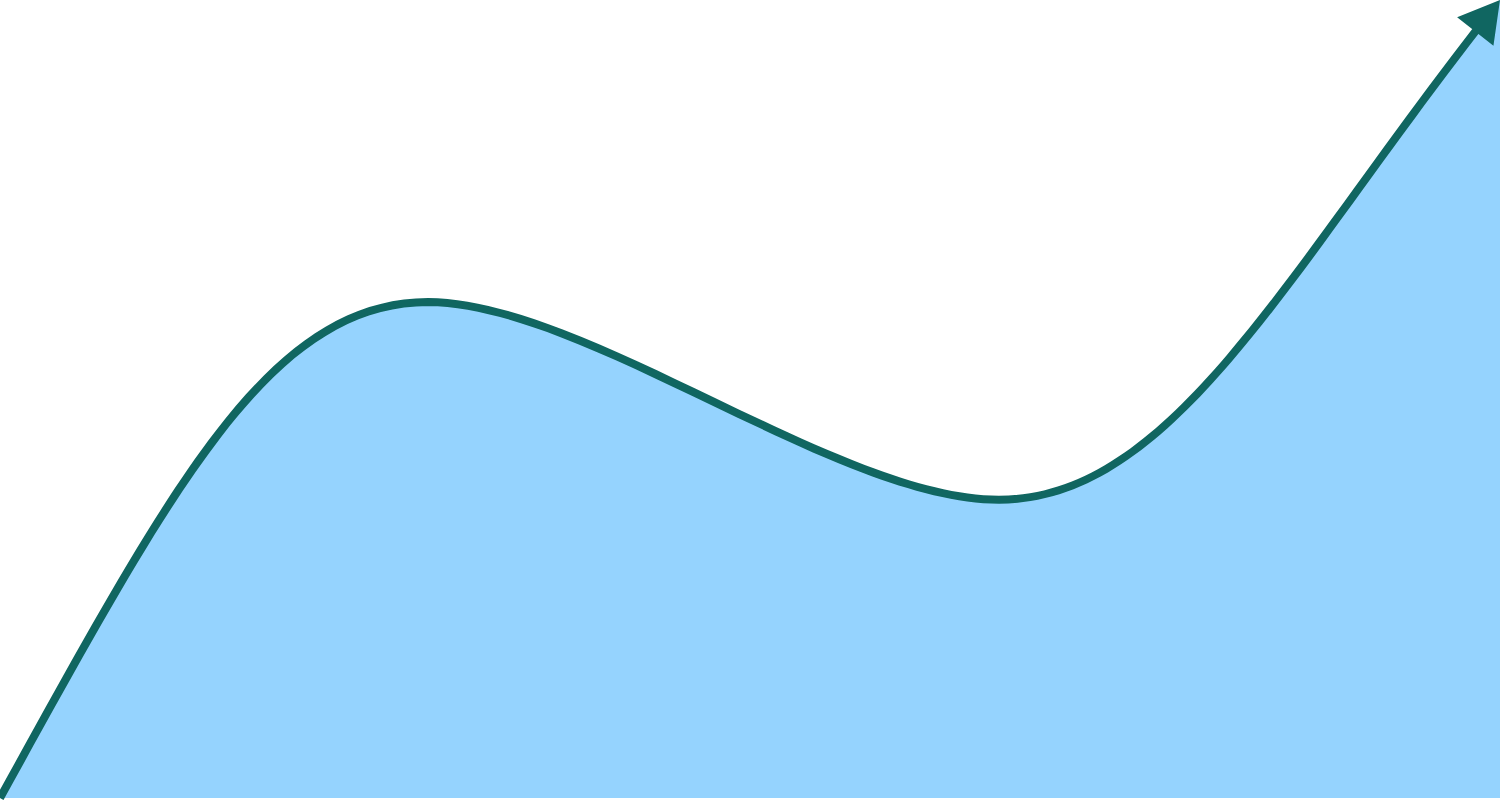# Problems of the Week

Contribute a problem

Given any polygon with perimeter 1, can a circle with radius $\frac14$ always enclose the polygon?Do there exist 3 distinct positive integers $(x, y, z )$ such that $x+y$, $y+z$, $z+x$, and $x + y + z$ are all perfect squares?A circular disk of radius $R$ consists of two uniform halves of equal area with masses $\frac{2}{3}M$ and $\frac{1}{3}M$. The disk is free to rotate on an axle through its geometric center.

Initially, the disk is at rest as shown on the left such that the half of mass $\frac23 M$ is on top.

Suppose the disk is toppled by an extremely gentle nudge. At the instant the disk first reaches the orientation on the right, its angular speed can be expressed as follows:

$\omega = \sqrt{\frac{Ag}{B \pi R}}.$

If $A$ and $B$ are coprime positive integers, determine $A + B$.

There exists a unique, positive-valued, non-constant, continuous and differentiable function $y = f(x)$ such that

• over any specified interval, the area between $f(x)$ and the $x$-axis is equal to the arc length of the curve, and
• $f(0) = 1.$

If $\displaystyle \int_{\ln2}^{\ln5} f(x) \, dx = \dfrac{a}{b}$, where $a$ and $b$ are coprime positive integers, then find $a + b$.Find the number of permutations $f$ on $\{1, 2, 3, \ldots, 32\}$ such that if $m$ divides $n$, then $f(m)$ divides $f(n)$.

×